Next: Sonic Flow through a Up: One-Dimensional Compressible Inviscid Flow Previous: Bernoulli's Theorem

# Mach Number

In an ideal gas, the local Mach number of the flow is defined (see Section 1.17)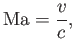(14.57)

where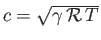is the local sound speed. [See Equation (14.45).] Setting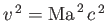in Equation (14.55), we obtain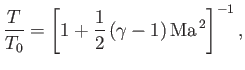(14.58)

where use has been made of Equations (14.15) and (14.24), which imply that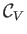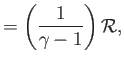(14.59)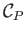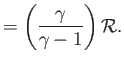(14.60)

Incidentally, the relation (14.58) is valid for any streamline, because the stagnation temperature,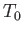, can be defined, by means of Equation (14.55), even when the streamline in question does not pass through a stagnation point. We can combine Equation (14.58) with the isentropic relation,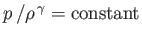along a streamline (see Section 14.3), as well as the ideal gas law, (14.1), to give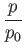(14.61)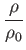(14.62)

Here,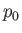and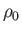are the pressure and density, respectively, at the stagnation point. In principle, the stagnation values,,, and, can be different on different streamlines. However, if a solid object moves through a homogeneous ideal gas that is asymptotically at rest then the stagnation parameters become true constants, independent of the streamline. Such flow is said to be homentropic.

A point where the speed of a steadily flowing ideal gas equals the local speed of sound,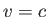, is termed a sonic point. The sonic temperature,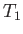, pressure,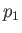, and density,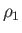, are simply related to the stagnation values. In fact, setting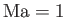in Equations (14.58), (14.61), and (14.62), we obtain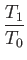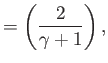(14.63)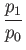(14.64)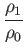(14.65)

Finally, if we combine Equations (14.58), and (14.61)-(14.65), then we get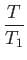(14.66)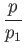(14.67)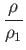(14.68)Next: Sonic Flow through a Up: One-Dimensional Compressible Inviscid Flow Previous: Bernoulli's Theorem
Richard Fitzpatrick 2016-01-22